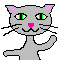Spreadsheets: A Dynamic Path to Understanding Mathby Wendy PettiSpreadsheets: A Dynamic Path to Understanding MathThe information, spreadsheet files, and other resources below are intended to support the NCTM session: "Spreadsheets: A Dynamic Path to Understanding Math."            Discussion Forum for Spreadsheets: Magic Beans from Roots to Clouds Spreadsheet SamplesPlease right-click the link below and save the Excel file to your computer. The file contains many sample spreadsheet activities and suggestions and a page of "big ideas" and supporting information.            spreadsheets-path-to-math-Petti.xls - updated October 15, 2010            cats-puzzle-locked.xls     This second spreadsheet is a little password-protected bonus.Solve the puzzle of the cats on the bus from the first spreadsheet to reveal the numerical password to open this spreadsheet.            cats-puzzle-solution.xls Give up? This variation shows the cats puzzle solution without password protection.            birthdays.xls - (For PC versions of Excel only.)This bonus "birthdays" spreadsheet uses a Visual Basic macro to generate random sortings of several thousand celebrity birthdays and checks to see if there are any matching birthdays among the first 23 celebrities in each random sort. (How often do you think there will be a match? Predict, then investigate!) Spreadsheet Activity Ideas and Instructions Topics and activities covered in the spreadsheet sampler include: extending patterns with click-and-drag to create/explore arithmetic and geometric sequences using formulas to create/explore arithmetic, geometric, and special sequences: triangular, square, cube, Fibonacci linking formulas to explore the "grains of rice" story and compound interest using formulas for measurement, money, time conversions, and instant-summing magic squares sorting lists to alphabetize or organize by numerical value illustrating real-life data with bar, double bar, line, pie, and/or pictographs using sliders and hidden formulas to create function machine puzzles using sliders to create dynamic graphs (with lines or curves that move as the slider value changes): linear, quadratic, cubic, and sine using random number generation and the frequency function, combined with a graph, to produce instant sets of 30 or more random rolls of dice pairs using random number generation to create math bingo boards using the average, median, mode, min and max functions to analyze data using conditional functions to create a self-checking quiz (such as estimating the sum of four random 3-digit numbers) using conditional formatting in creating simple math games discovering the square root of any number with two methods (guess-and-check and Babylonian/Newton) analyzing student growth data using filtering, sorting, graphing, functions filtering a checklist of qualities of geometric shapes to identify terms sharing common features combining autoshapes and other drawing tools with angled text to create geo-messages using a "brick wall" merge pattern and formulas to explore Pascal's triangle and its many properties using the comment feature combined with graphics to create pop-up mini-lessons using internal hyperlinks to create tutorials or interactive math stories creating a game or puzzle sequence with levels by using the solution of each puzzle as the password to open the new level in a different password-protected workbook. Why use spreadsheets in the math program? Spreadsheets are a powerful tool for exploring and constructing meaning in dynamic and creative ways. Students can experience elementary algebra in action as they create and use formulas and function tables. The patterns in a multiplication grid take on new meaning when students can extend a core 2 x 2 grid to any multiple with a few swipes of a mouse. Probability experiments can be instantly analyzed with dynamic graphs that adjust automatically as new data is entered. Students can make predictions and formulate conjectures as they compare theoretical data with real-life data. Spreadsheets empower students as they facilitate inquiry and analysis. Supporting Research Extensive research supports the use of spreadsheets in mathematics classrooms to empower student-centered learning. J. C. Russell, in Spreadsheet Activities in Middle School Mathematics (NCTM, 1992), observed that a blank spreadsheet can be seen as a "student of the student," capable of being taught, as directed by the student. The act of teaching the computer to do something specific and meaningful with data helps a student to clarify his or her own understanding of the problem. Useful quotes and references are cited in Using Spreadsheets in Mathematics Education, ERIC Clearinghouse for Math, Science, and Environmental Education (Columbus, Ohio, 2000 - www.ericdigests.org/2003-1/math.htm). The NCTM's 2000 Principles and Standards recommend that "students in grades 3-5 should also become familiar with technological tools such as dynamic geometry software and spreadsheets. They should learn to set up a simple spreadsheet and use it to pose and solve problems, examine data, and investigate patterns" (NCTM, 2000, p. 207). Alex Friedlander, in "An EXCELlent bridge to algebra" (Mathematics Teacher, 1998, 91(50), 382-383), states: "Spreadsheets build an ideal bridge between arithmetic and algebra and allow the student free movement between the two worlds. Students look for patterns, construct algebraic expressions, generalize concepts, justify conjectures, and establish the equivalence of two models as intrinsic and meaningful needs rather than as arbitrary requirements posed by the teacher."

______________________________

Do you have ideas, resources, or good questions to share?
I welcome your input!

 [PLEASE ENABLE JAVASCRIPT TO SEE THIS][PLEASE ENABLE JAVASCRIPT TO SEE THIS]

 © copyright 2008 -   Wendy Petti of Math Cats.   All Rights Reserved.